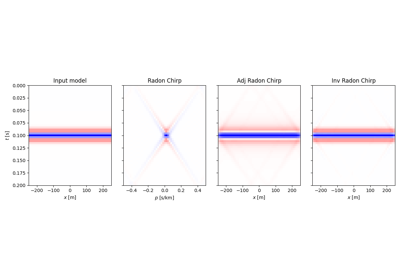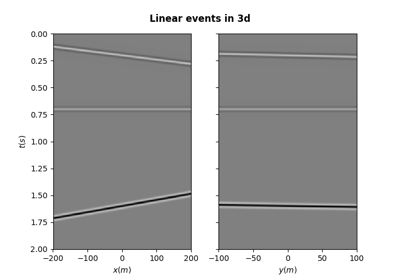# pylops.utils.seismicevents.linear3d#

pylops.utils.seismicevents.linear3d(x, y, t, v, t0, theta, phi, amp, wav)[source]#

Linear 3D events

Create 3d linear events given propagation velocity, intercept time, angles, and amplitude of each event.

Parameters
xnumpy.ndarray

space axis in x direction

ynumpy.ndarray

space axis in y direction

tnumpy.ndarray

time axis

vfloat

propagation velocity

t0

intercept time at $$x=0$$ of each linear event

theta

angle in x direction (in degrees) of each linear event

phi

angle in y direction (in degrees) of each linear event

amp

amplitude of each linear event

wavnumpy.ndarray

wavelet to be applied to data

Returns
dnumpy.ndarray

data without wavelet of size $$[n_y \times n_x \times n_t]$$

dwavnumpy.ndarray

data with wavelet of size $$[n_y \times n_x \times n_t]$$

Notes

Each event is created using the following relation:

$t_i(x, y) = t_{0,i} + p_{x,i} x + p_{y,i} y$

where $$p_{x,i}=\frac{1}{v} \sin( \theta_i)\cos( \phi_i)$$ and $$p_{x,i}=\frac{1}{v} \sin( \theta_i)\sin( \phi_i)$$.

## Examples using pylops.utils.seismicevents.linear3d#